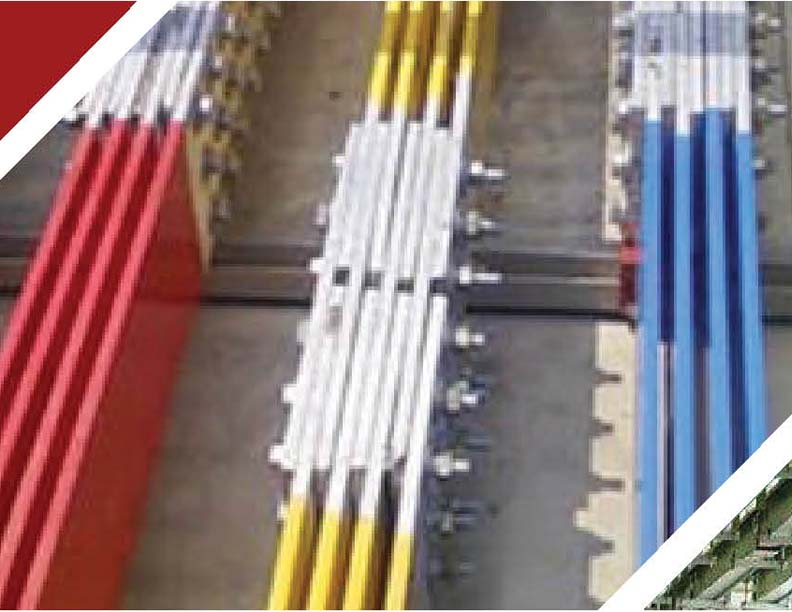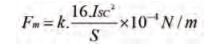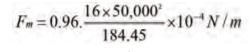# BUSDUCT SYSTEM DESIGN Part 3

In order to have low losses and enhanced reliability in the distribution system, bus-ducts are preferred over cables for higher current ratings and shorter lengths at different voltage levels. Though Sandwich Bustrunking System with rated operational voltage (Ue) 1000 Volt are easily available, when it comes to economics–Air insulated bus ducts have an edge. This article focuses on the types of air insulated busducts and their selection guidelines, design requirements, de-rating factors and sizing requirement...The reactance thus obtained can be doubled for single phase systems. For a three phase system the configuration of the three phases with respect to each other will play a significant role and the linear centre spacing S has to be modified to an effective or geometric mean spacing Se.

Se=(Sa.Sb.Sc)1/3  for the configuration shown in figure-10

Sa = Sb = S

Sa+ Sb = Sc

Sc = 2S

Se = S. 21/3 = 1.26 S

Example-4:

Considering example-3, for determining the effect of proximity

a/b = 44.45/101.6 = 0.4375

1.26 * S / (a+b) = 1.59 then Xa due to the proximity affect from the graph = 125*10-6 ohm/m = 0.125 ohm/ 1000m per phase Impedance,Voltage drop considering a length of busbar as 20m and current rating as 4000A

= 40000.12620/10000 = 10.08V which is 2.43% for 415V system which is acceptable

Proximity effect on the enclosure:

The electric field produced by the current carrying conductors of each phase in addition links the metallic bus enclosure, its mounting supports and its structures existing in the vicinity, parallel and around the axis of the current carrying conductors. It causes induced currents in such structures which therefore leads to resistance and magnetic losses.

The electrodynamic forces between the enclosure and the conductors will be small because the enclosure, which is non-continuous, will carry much less current when compared to conductors. They therefore need not be considered separately, as the metallic structure will have sufficient strength to bear them.

In a non-magnetic enclosure, such as Aluminium or stainless steel, only resistance losses occur. In a magnetic enclosure, such as mild steel, there will also be hysteresis and eddy current losses in addition to resistance losses. All these losses appear as heat in the enclosure and the metallic structure. Depending upon the current rating, the configuration of the busbars, the choice of the material of the enclosure should be such that it minimizes these effects as far as possible. Since the spacing in an HT system is already large, an HT system generally not effected by the proximity effects.

Minimizing the proximity effect:

Maintaining greater spacing between the phases

This can be achieved by providing adequate clearances between the conductors and inside the enclosure. In thicker sections or where a number of smaller sections are used together to form a phase, the current will accumulate at the outer surfaces only rather than the centre. Therefore, to achieve an almost zero proximity effect condition, it is desirable to provide a space of 300mm and more between the extreme outer surfaces, rather than between centres. But to keep the phase conductors completely out of inductive effect of the other phases, it may require very large enclosures, particularly at higher ratings (above 2000A) which may not be practical.

Phase interleaving

This is highly efficient and more practical method for large ratings and offers the utilization of conductors. It provides an almost balanced and a low reactance system. Each phase, comprising of a number of conductors, is split into two or more groups. It is however suggested to limit the number of groups to only two taking into account of size and the cost of enclosure. In four conductors, two groups each with two conductors per phase can be arranged as shown in figure-11 to establish a low reactance system as a result of the smaller spacings between the split phases on the one hand, and two parallel paths on the other. The two parallel paths will further reduce the total reactance to one half. The field produced by each split phase would now become half and fall out of the inductive region of the other. The arrangement would thus provide a system with a low proximity effect.

Since the conductors in each phase are now arranged as close as dielectrically possible, the electrodynamic forces on each group in the event of fault on considering the spacing would be high as a result of the smaller spacing between the phase conductors. But the overall forces would become much less compared to the conventional arrangement because of two or more parallel current paths, each carrying a reducing amount of current, depending upon the number of parallel paths so formed. Moreover, the mounting supports will also become stronger than before, because there are as many mounting supports as the number of parallel paths, each sharing the total force equally. The method of interleaving will therefore require no additional reinforcement of the busbar supports or the mounting structures. As a result of the low reactance obtained the arrangement will provide a quite inductively balanced system. The reactance of the conductors can be calculated on an individual group basis and then halved when the conductors are split into two halves.

The arrangement will also minimize the skin effect to a very great extent, as the current of each phase is now shared by two or more independent circuits, each of a thinner section than a composite phase.

Example-5: Considering example 2, using four sections of 101.6 x 6.35mm Al conductors, now interleaved as shown in figure-12., the improved reactance and resistance would be?.

a/b = 0.1875 and space factor = 1.28 x S / (a+b) = 0.98 Xa from graph of figure-9 = 90 x 10-6ohm/m and for two parallel circuits = 90/2 = 45 x 10-6ohm/m or 0.045 ohm/1000m as against 125×10-6ohm/m with the conventional arrangement

Skin effect: Area of cross section per split phase = 2×101.6×6.35 = 12.9 cm2

b/a = 101.6/19.05 = 5.33

from the graph Rac/Rdc = 1.13

Rdc = 0.056 ohm / 1000m per conductor for Rdc = 0.056/4 = 0.014 ohm /1000m per 4 conductors

Rac = 0.014 x 1.13 = 0.158 ohm/1000mVoltage drop for 20m of bus length

= 2000 x 0.0477 x 20 /1000 = 3.82V which is even less

than 1% for a 415V bus system.

Electrodynamic forces:

For a system fault level of 50kA maximum forces oneach groupFor conventional arrangement:

K for a space factor of S-a/a+b = (184.45-44.45) / (44.45+101.6) = 0.958 corresponding to a/b = 0.4375 from the graph is K = 0.96Fm = 20819 N/m

For the improved interleaving arrangement:

K for a space factor of S-a/a+b = (94.45-19.05) / (19.05+101.6) = 0.62 corresponding to a/b = 0.185 from the graph is K = 0.87Fm = 9250.4 N/m

Fm on both supports = 2 x 9250.4 = 18500.8 N/m

This is less than the force developed with the conventional arrangement.Chanukya Annepu

The author is from the Engineering Services Division, Indira Gandhi Centre for Atomic Research, Kalpakkam, India